## 3.10.1 Non-Equilibrium GREEN's Function

Throughout this chapter the NEGF formalism has been described. GREEN's functions in general have two position and two time arguments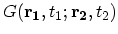. Under steady-state condition one can FOURIER transform the time difference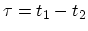to energy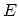to obtain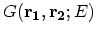(Section 3.8.3), which can further be transformed via the WIGNER-WEYL procedure into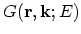.

The NEGF method addresses the problem of dissipative quantum transport in a consistent and complete way. Therefore, the method is computationally expensive and applied to systems in a steady-state . The quantum device region is coupled to the reservoirs by contact self-energies (Appendix G), while dissipation is introduced via the scattering self-energies.

When scattering via a self-energy is introduced, the determination of the GREEN's function requires inversion of a matrix of huge rank. To reduce the computational cost, the local scattering approximation is frequently used [208,112]. In this approximation the scattering self-energy terms are diagonal in coordinate representation. It allows one to employ the recursive algorithm for computing the GREEN's functions, see Appendix H [116,8]. The local approximation is well justified for electron-phonon scattering caused by deformation potential interaction (Section 4.6). However, it is not justified for scattering by polar optical phonons, surface roughness, and ionized impurities.

In order to reduce the computational cost even further, systems with simplified grid requirements are considered. The mode-space approach (Section 4.4)  takes only a relatively small number of transverse modes,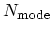, into consideration. For the remaining, one-dimensional transport problem the number of unknowns reduces to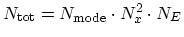, where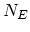is the number of energy grid points.

It can be shown that the quantum ballistic formalism can be fully recovered from the NEGF formalism as a special case, where no dissipative scattering occurs in the system . Another important point is that the NEGF formalism looks very different from the LANDAUER-BÜTTIKER formalism  [209,210]. The NEGF formalism focuses on the internal state of the conductor. By contrast, in the LANDAUER approach the central quantity is the transmission function from one contact to another. The internal state of the conductor usually never appears in this formalism. However, the transmission function can be expressed in terms of internal quantities. One can precisely obtain this result from the NEGF formalism as well when non-dissipative transport is assumed.

M. Pourfath: Numerical Study of Quantum Transport in Carbon Nanotube-Based Transistors